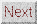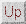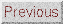# waynehu

## Professor, Department of Astronomy and Astrophysics University of Chicago

Group Contact CV SnapShots

While a review of the standard cosmological paradigm is not our intention (see [Narkilar & Padmanabhan, 2001] for a critical appraisal), we briefly introduce the observables necessary to parameterize it.

The expansion of the Universe is described by the scale factor a(t), set to unity today, and by the current expansion rate, the Hubble constant H0=100h km/s/Mpc with h~0.7 [Freedman et al, 2001]. The Universe is flat (no spatial curvature) if the total density is equal to the critical density, &rhoc=1.88h2x 10-29 g/cm3; it is open (negative curvature) if the density is less than this and closed (positive curvature) if greater. The mean densities of different components of the Universe control a(t) and are typically expressed today in units of the critical density &Omegai, with an evolution with a(t) specified by equations of state wi= pi/&rhoi, where pi is the pressure of the ith component. Density fluctuations are determined by these parameters through the gravitational instability of an initial spectrum of fluctuations.

The working cosmological model contains photons , neutrinos , baryons , cold dark matter , and dark energy with densities proscribed within a relatively tight range. For the radiation, &Omegar=4.17x10-5h-2 (wr=1/3). The photon contribution to the radiation is determined to high precision by the measured CMB temperature, T=2.728±0.004K [Fixsen et al, 1996]. The neutrino contribution follows from the assumption of 3 neutrino species, a standard thermal history, and a negligible mass m&nu <<1eV. Massive neutrinos have an equation of state w&nu=1/3→0 as the particles become non-relativistic. For m&nu~1eV this occurs at a~10-3 and can leave a small but potentially measurable effect on the CMB anisotropies [Ma & Bertschinger, 1995,Dodelson et al, 1996].

For the ordinary matter or baryons, &Omegab~0.02h-2 (wb~0) with statistical uncertainties at about the ten percent level determined through studies of the light element abundances (for reviews, see [Boesgaard & Steigman, 1985,Schramm & Turner, 1998,Tytler et al, 2000]). This value is in strikingly good agreement with that implied by the CMB anisotropies themselves as we shall see. There is very strong evidence that there is also substantial non-baryonic dark matter. This dark matter must be close to cold (wm=0) for the gravitational instability paradigm to work [Peebles, 1982] and when added to the baryons gives a total in non-relativistic matter of &Omegam~1/3. Since the Universe appears to be flat, the total &Omegatot must be equal to one. Thus, there is a missing component to the inventory, dubbed dark energy, with &Omega&Lambda ~ 2/3. The cosmological constant (w&Lambda=-1) is only one of several possible candidates but we will generally assume this form unless otherwise specified. Measurements of an accelerated expansion from distant supernovae [Riess et al, 1998,Perlmutter et al, 1999] provide entirely independent evidence for dark energy in this amount.

The initial spectrum of density perturbations is assumed to be a power law with a power law index or tilt of n ~1 corresponding to a scale-invariant spectrum. Likewise the initial spectrum of gravitational waves is assumed to be scale-invariant, with an amplitude parameterized by the energy scale of inflation Ei, and constrained to be small compared with the initial density spectrum. Finally the formation of structure will eventually reionize the Universe at some redshift 7 < z < 20

Many of the features of the anisotropies will be produced even if these parameters fall outside the expected range or even if the standard paradigm is incorrect. Where appropriate, we will try to point these out.Next: CMB Temperature Field Up: OBSERVABLES Previous: OBSERVABLES
Wayne Hu 2001-10-15